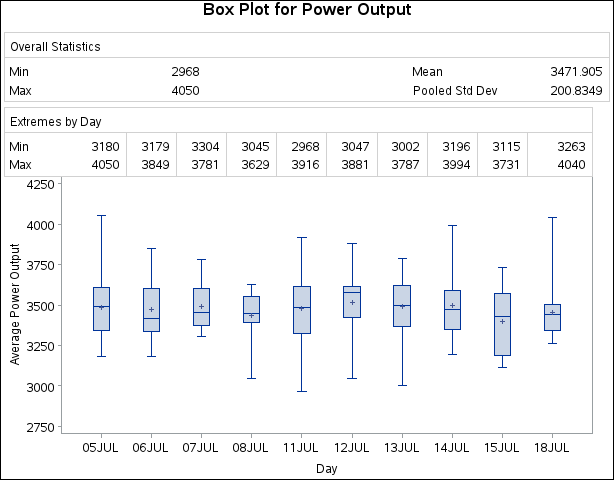Example 28.1 Displaying Summary Statistics in a Box Plot

This example demonstrates how you can use the INSET and INSETGROUP statements to include tables of summary statistics in your box plots. The following statements produce a box plot of the `Turbine` data set from the section Getting Started: BOXPLOT Procedure, augmented with insets containing summary statistics:

```ods graphics off;
title 'Box Plot for Power Output';
proc boxplot data=Turbine;
plot KWatts*Day;
inset min mean max stddev /
pos    = tm;
insetgroup min max /